# Biconditional Statements

## Biconditional Statement Problems With Interactive Exercises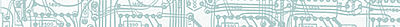Example 1: Examine the sentences below.

 Given: p: A polygon is a triangle. q: A polygon has exactly 3 sides. Problem: Determine the truth values of this statement: (p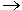q)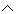(qp)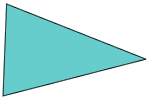The compound statement (pq)(qp) is a conjunction of two conditional statements. In the first conditional, p is the hypothesis and q is the conclusion; in the second conditional, q is the hypothesis and p is the conclusion. Let's look at a truth table for this compound statement.

 p q pq qp (pq)(qp) T T T T T T F F T F F T T F F F F T T T

In the truth table above, when p and q have the same truth values, the compound statement (pq)(qp) is true. When we combine two conditional statements this way, we have a biconditional.

Definition: A biconditional statement is defined to be true whenever both parts have the same truth value. The biconditional operator is denoted by a double-headed arrow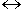. The biconditional pq represents "p if and only if q," where p is a hypothesis and q is a conclusion. The following is a truth table for biconditional pq.

 p q pq T T T T F F F T F F F T

In the truth table above, pq is true when p and q have the same truth values, (i.e., when either both are true or both are false.) Now that the biconditional has been defined, we can look at a modified version of Example 1.

Example 1:

 Given: p: A polygon is a triangle. q: A polygon has exactly 3 sides. Problem: What does the statement pq represent? Solution: The statement pq represents the sentence, "A polygon is a triangle if and only if it has exactly 3 sides."Note that in the biconditional above, the hypothesis is: "A polygon is a triangle" and the conclusion is: "It has exactly 3 sides." It is helpful to think of the biconditional as a conditional statement that is true in both directions.Remember that a conditional statement has a one-way arrow () and a biconditional statement has a two-way arrow (). We can use an image of a one-way street to help us remember the symbolic form of a conditional statement, and an image of a two-way street to help us remember the symbolic form of a biconditional statement.

Let's look at more examples of the biconditional.

Example 2:

 Given: a: x + 2 = 7 b: x = 5 Problem: Write ab as a sentence. Then determine its truth values ab.

Solution: The biconditonal ab represents the sentence: "x + 2 = 7 if and only if x = 5." When x = 5, both a and b are true. When x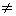5, both a and b are false. A biconditional statement is defined to be true whenever both parts have the same truth value. Accordingly, the truth values of ab are listed in the table below.

 a b ab T T T T F F F T F F F T

Example 3:

 Given: x: I am breathing y: I am alive Problem: Write xy as a sentence.

Solution: xy represents the sentence, "I am breathing if and only if I am alive."

Example 4:

 Given: r: You passed the exam. s: You scored 65% or higher. Problem: Write rs as a sentence.

Solution:  rs represents, "You passed the exam if and only if you scored 65% or higher."

Mathematicians abbreviate "if and only if" with "iff." In Example 5, we will rewrite each sentence from Examples 1 through 4 using this abbreviation.

Example 5: Rewrite each of the following sentences using "iff" instead of "if and only if."

 if and only if iff A polygon is a triangle if and only if it has exactly 3 sides. A polygon is a triangle iff it has exactly 3 sides. I am breathing if and only if I am alive. I am breathing iff I am alive. x + 2 = 7 if and only if x = 5. x + 2 = 7 iff x = 5. You passed the exam if and only if you scored 65% or higher. You passed the exam iff you scored 65% or higher.

When proving the statement p iff q, it is equivalent to proving both of the statements "if p, then q" and "if q, then p." (In fact, this is exactly what we did in Example 1.) In each of the following examples, we will determine whether or not the given statement is biconditional using this method.

Example 6:

 Given: p: x + 7 = 11 q: x = 5 Problem: Is this sentence biconditional?  "x + 7 = 11 iff x = 5."

Solution:

Let pq represent "If x + 7 = 11, then x = 5."
Let qp represent "If x = 5, then x + 7 = 11."

The statement pq is false by the definition of a conditional. The statement qp is also false by the same definition. Therefore, the sentence "x + 7 = 11 iff x = 5" is not biconditional.

Example 7:

Given:

r: A triangle is isosceles.

s: A triangle has two congruent (equal) sides.

Problem:

Is this statement biconditional?  "A triangle is isosceles if and only if it has two congruent (equal) sides."Solution: Yes. The statement rs is true by definition of a conditional. The statement sr is also true. Therefore, the sentence "A triangle is isosceles if and only if it has two congruent (equal) sides" is biconditional.

Summary: A biconditional statement is defined to be true whenever both parts have the same truth value. The biconditional operator is denoted by a double-headed arrow. The biconditional pq represents "p if and only if q," where p is a hypothesis and q is a conclusion.

### Exercises

Directions: Read each question below. Select your answer by clicking on its button. Feedback to your answer is provided in the RESULTS BOX. If you make a mistake, choose a different button.

1.
 Given: a: y - 6 = 9 b: y = 15 Problem: The biconditional a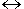b represents which of the following sentences?

If y - 6 = 9, then y = 15.
y - 6 = 9 if and only if y = 15.
If y = 15, then y - 6 = 9.
None of the above.

RESULTS BOX:

2.
 Given: r: 11 is prime. s: 11 is odd. Problem: The biconditional rs represents which of the following sentences?

If 11 is prime, then 11 is odd.
If 11 is odd, then 11 is prime.
11 is prime iff 11 is odd.
None of the above.

RESULTS BOX:

3.
 Given: xy yx Problem: If both of these statements are true then which of the following must also true?

(xy)(yx)
xy
x iff y
All of the above.

RESULTS BOX:

4.
 Given: mn is biconditional Problem: Which of the following is a true statement?

m is the hypothesis
m is the conclusion
n is a conditional statement
n is a biconditional statement

RESULTS BOX:

 5. Which of the following statements is biconditional? I am sleeping if and only if I am snoring.Mary will eat pudding today if and only if it is custard.It is raining if and only if it is cloudy.None of the above. RESULTS BOX:
 Lessons on Symbolic Logic Negation Conjunction Disjunction Conditional Compound Biconditional Tautologies Equivalence Practice Exercises Challenge Exercises Solutions